# Part A: 10 points each (Questions 1-4 1. A block mass of 3 kg attached with a spring kg attached with a spring of s...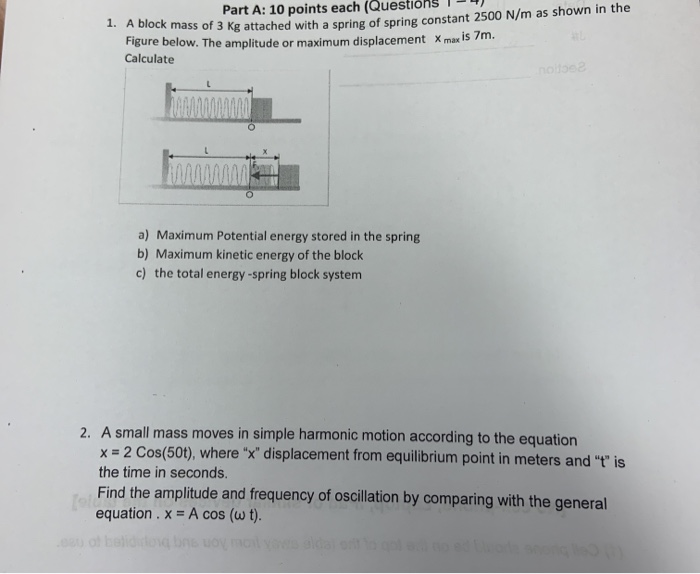Part A: 10 points each (Questions 1-4 1. A block mass of 3 kg attached with a spring kg attached with a spring of spring constant 2500 N/m as shown in the Figure below. The amplitude or maximum displacement X max is 7m. Calculate O a) Maximum Potential energy stored in the spring b) Maximum kinetic energy of the block c) the total energy-spring block system 2. A small mass moves in simple harmonic motion according to the equation x = 2 Cos(50t), where "x" displacement from equilibrium point in meters and "t" is the time in seconds. Find the amplitude and frequency of oscillation by comparing with the general equation . x = A cos (w t).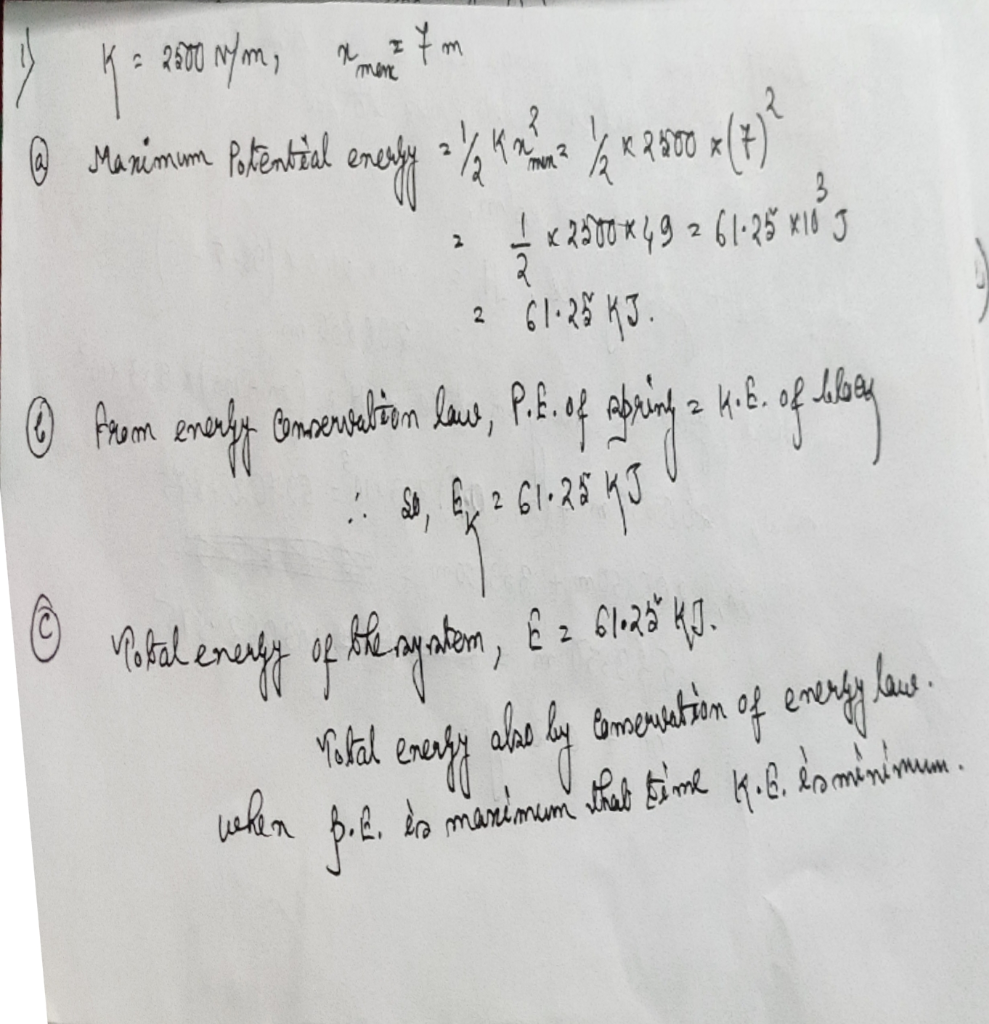##### Add Answer of: Part A: 10 points each (Questions 1-4 1. A block mass of 3 kg attached with a spring kg attached with a spring of s...
Similar Homework Help Questions
• ### 1. A simple harmonic motion of an object of mass m = 11 kg attached with a spring is represented as time vs displac...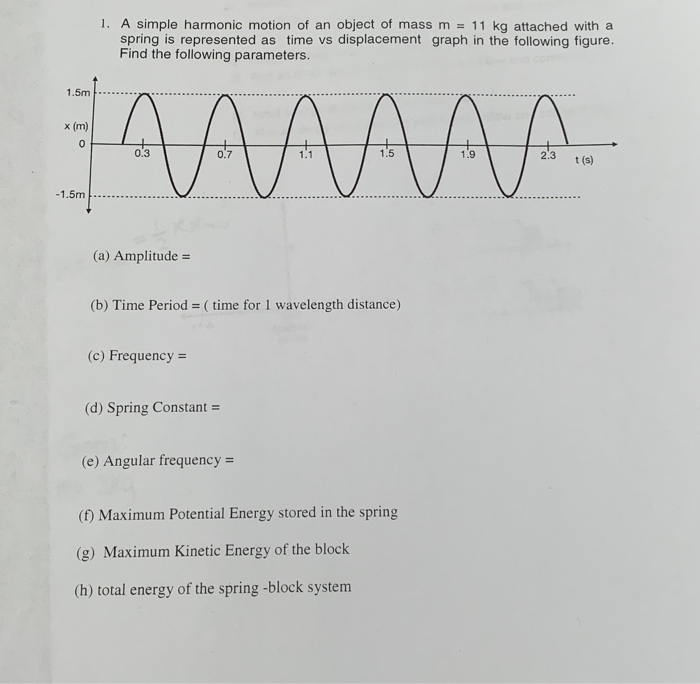1. A simple harmonic motion of an object of mass m = 11 kg attached with a spring is represented as time vs displacement graph in the following figure. Find the following parameters. 1.5m - АААААА 0.3 23 23 tis) -1.5m (a) Amplitude = (b) Time Period = (time for 1 wavelength distance) (c) Frequency = (d) Spring Constant = (e) Angular frequency = (f) Maximum Potential Energy stored in the spring (g) Maximum Kinetic Energy of the block (h)...

• ### 1. A simple harmonic motion of an object of mass m = 11 kg attached with...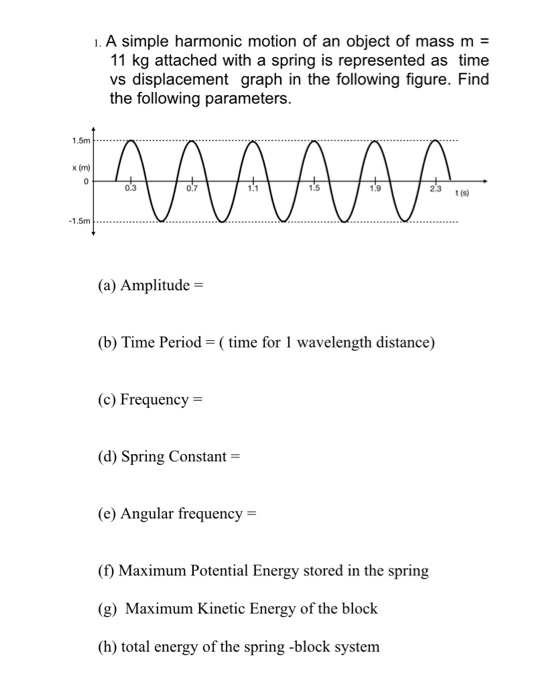1. A simple harmonic motion of an object of mass m = 11 kg attached with a spring is represented as time vs displacement graph in the following figure. Find the following parameters. ТАЛААР (a) Amplitude = (b) Time Period =( time for 1 wavelength distance) (c) Frequency = (d) Spring Constant = (e) Angular frequency = (1) Maximum Potential Energy stored in the spring (g) Maximum Kinetic Energy of the block (h) total energy of the spring -block system

• ### 1. A simple harmonic motion of an object of mass m = 11 kg attached with...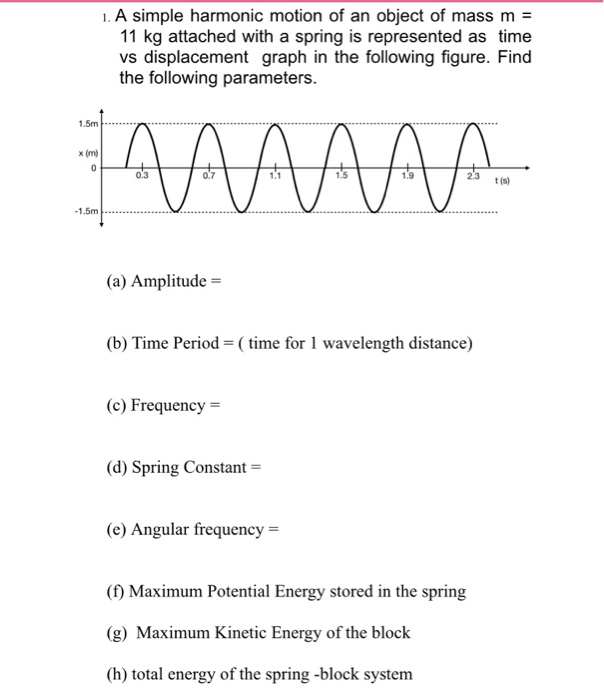1. A simple harmonic motion of an object of mass m = 11 kg attached with a spring is represented as time vs displacement graph in the following figure. Find the following parameters. (a) Amplitude = (b) Time Period = ( time for 1 wavelength distance) (c) Frequency = (d) Spring Constant = (e) Angular frequency = (f) Maximum Potential Energy stored in the spring (g) Maximum Kinetic Energy of the block (h) total energy of the spring -block system

• ### Problem3 A (2+0.1y) kg block attached to a spring undergoes simple harmonic motion described by x...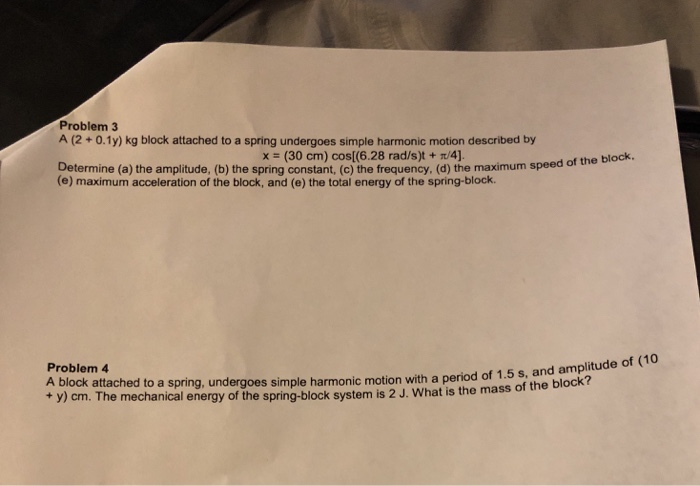Can you please answer both questions, Y=0 Problem3 A (2+0.1y) kg block attached to a spring undergoes simple harmonic motion described by x (30 cm) cos[(6.28 rad/s)t + /4) Determine (a) the amplitude, (b) the spring constant, (c) the frequency, (d) the maximum speed (e) maximum acceleration of the block, and (e) the total energy of the spring-block. of the block Problem 4 A block attached to a spring, undergoes simple harmonic motion with a period of 1.5 + y)...

A block of mass m = 12 kg is attached to one end of a massless, horizontal spring of spring constant k = 100N/m. The other end of the spring is fixed.The mass executes simple harmonic motion with a maximum displacement ofxmax = 0.6 m from equilibrium. A quantity of putty of mass mp = 4 kg is then droppedonto the oscillating block and adheres to it.a) What are the initial period of oscillation T0, amplitude of oscillation A0, maximum...

• ### Mass on a Spring

Please show work and explain, thank you!A block of mass m = 12 kg is attached to one end of a massless, horizontal spring of spring constant k = 100 N/m. The other end of the spring is ﬁxed. The massexecutes simple harmonic motion with a maximum displacement of xmax = 0.6 m from equilibrium. A quantity of putty of mass mp = 4 kg is thendroppedonto the oscillating block and adheres to it.a) What are the initial period of...

• ### Ignore damping forces. A mass of 4 kg is attached to a spring with constant k- 16 N/m, then the s...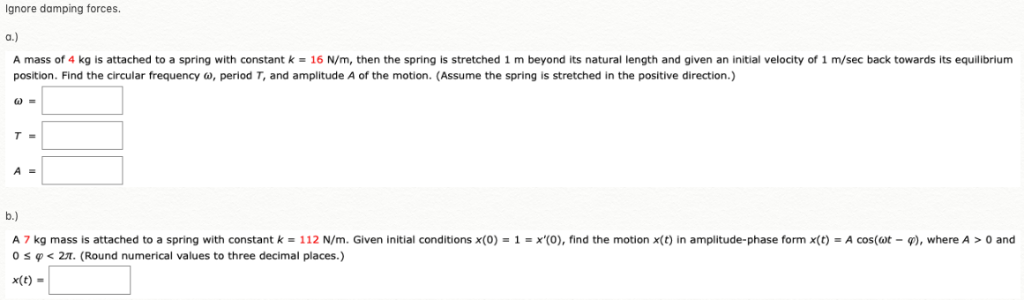Ignore damping forces. A mass of 4 kg is attached to a spring with constant k- 16 N/m, then the spring is stretched 1 m beyond its natural length and given an initial velocity of 1 m/sec back towards its equilibrium position. Find the circular frequency ω, period T, and amplitude A of the motion. (Assume the spring is stretched in the positive direction.) A 7 kg mass is attached to a spring with constant k 112 N m. Given...

• ### A simple harmonic oscillator consists of a block of mass 1.60 kg attached to a spring of spring c...

A simple harmonic oscillator consists of a block of mass 1.60 kg attached to a spring of spring constant 170 N/m. When t = 1.50 s, the position and velocity of the block are x = 0.126 m and v = 3.090 m/s. (a) What is the amplitude of the oscillations? What were the (b) position and (c) velocity of the block at t = 0 s?

• ### A horizontal spring with spring constant of 9.80 N/m is attached to a block with a mass of 1.20...

A horizontal spring with spring constant of 9.80 N/m is attached to a block with a mass of 1.20 kg that sits on a frictionless surface. When the block is 0.345 m fromits equilibrium position, it has a speed of 0.540 m/s.(a) What is the maximum displacement of the block from the equilibrium position?m(b) What is the maximum speed of the block?m/s(c) When the block is 0.200 m from the equilibrium position, what is its speed?m/s

• ### A 1.0 kg block is attached to a spring with spring constant 16 N/m

A 1.0 kg block is attached to a spring with spring constant 16 N/m. While the block is sitting at rest, a student hits it with a hammer and almost instantaneously gives it a speed of 40cm/s. What are a: the amplitude of the subsequent oscillations? b: the block's speed at the point where x=1/2A

Need Online Homework Help?